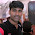Problems based on Ages

Today I'm going to discuss some problems based on ages. These are an important questions regarding bank exams and other competitive exams. To solve the problems related to ages, You will require knowledge of linear equations. I have tried to evaluate some easy and quick method for you people to solve these types of questions.

Some important points

• Assume the present ages, then proceed with the equations.
• While making equations, always remember to convert both sides into either present year or the given year i.e. time frame should be same.
• 5 years ago topresent year - 5 years
• 5 years hence to present year + 5 years
Problems with Solution

Consider the following example:

Example: The age of father 2 years ago 7 times the age of his son. At present, fathers age is five times that of his son . What are the ages of father and son at present?

Solution: Let present age of son = x
present age of father = y

 Age 2 years ago Present age Son x - 2 x Father 7(x-2) y = 5x

Then read the question and write the given equations.

Last step, Just make an equation using values of father's age.

Either, convert it both into present ages or 2 years ago

2years ago,  7(x-2) = 5x-2.............................................. 5x is present age of father. I converted it into age before 2 years

So equation is, 7(x-2) = (5x-2)

Solving equation, x =6 yrs
Therefore, present age of son = 6yrs and
present age of father = 5 times 6 = 30 yrs

Example2: The age of father after 2 years is  4 times the age of his son. At present, father's age is five times that of his son . What are the ages of father and son at present?

Solution: The difference between both of examples is just ,one is 2 yrs ago and other is 2yrs hence.

Let present age of son = x yrs and
present age of father = y yrs

 Present age Age after 2 years Son x x+2 Father y = 5x 4(x+2)

Similarly, make an equation using values of father's age.

Either, convert it both into present ages or 2 years ago

2years hence,  4(x+ 2) = 5x+2.............................................. 5x is present age of father. I converted it into age after 2 years

So equation is, 4(x+2) = (5x+2)

Solving equation, x =6 yrs
Therefore, present age of son = 6yrs and
present age of father = 5 times 6 = 30 yrs.

Example3: 2 yrs earlier father's age was  7 times the age of his son. 2 years hence father's age would be 4 times the age of his son. What are the present ages of father and son?

Solution: Let present age of son = x yrs and
present age of father = y yrs

 Age 2 years ago Present age Age 2 years hence Son x – 2 x x+2 Father 7(x-2) y 4(x+2)

Making an equation using values of father's age.

2years ago,  7(x-2) = 4(x+2) -4
Here,4(x+2) is age after two years.Therefore, I converted it into age before 2 years by subtracted 4 years.

So equation is, 7(x-2) =4(x+2)-4

Solving equation, x =6 yrs
Therefore, present age of son = 6yrs and
present age of father = 7(x-2) + 2 (i.e. age before 2 years + 2 = present age)

⇒ 7(4) + 2 = 28 +2 = 30yrs.

Shortcut of these questions:

 Age 2 years ago Present age Age 2 years hence Father’s age as compared to son 7 5 4

1)  2 times (7-1)/(7-5)

2)   2 times (4-1)/(5-4)

3)  (2 times (7-1) + 2times (4-1))/ (7-4)

Shortcut of these three examples are very simple. Must try it once as it saves your time in exams. You should definitely try this once. But if you are not comfortable then you can do with the previous method.

Also check: Tricks of ratio and proportions

What's trending in BankExamsToday

Smart Prep Kit for Banking Exams by Ramandeep Singh - Download here

Join 40,000+ readers and get free notes in your email1.one more shortcut, which is applicable for all age problems.

Ex 1:
Ratio of father : son
2 years ago 7 : 1 (here difference is 7-1=6)
Present 5 : 1 (here difference is 5-1=4)

to make same difference, multiply 4 with 7 : 1 and 6 with 5 : 1
Now their ages becomes,
2 years ago 28 : 4
Present 30 : 6

1.what if the difference is same

2.3.in first example i think it need a correction please correct that one ------let us assume age of son is "x" 2 years ago at that time father's age is 7x,,,,,at present ages of son and father are x+2, 5(x+2),,,,,,,from that statement given we can equalize.........5(x+2)=5x we can solve and we get x=5 that means 2 years ago now 5+2=7years fathers age 5*7=35

I will try to respond asap